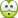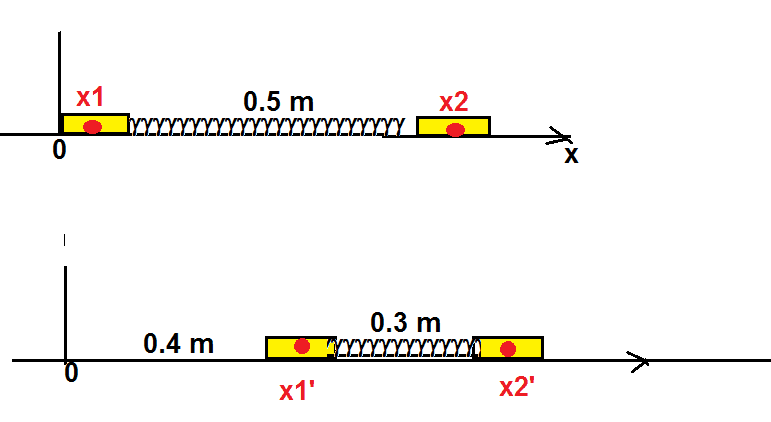# Two identical 0.50-kg carts, each 0.10 m long, are at rest on a low-friction track

Edel Crine
Homework Statement:
Two identical 0.50-kg carts, each 0.10 m long, are at rest on a low-friction track and are connected by a spring that is initially at its relaxed length of 0.50 m and is of negligible inertia. You give the cart on the left a push to the right (that is, toward the other cart), exerting a constant 5.0-N force. You stop pushing at the instant when the cart has moved 0.40 m. At this instant, the relative velocity of the two carts is zero and the spring is compressed to a length of 0.30 m. A locking mechanism keeps the spring compressed, and the two carts continue moving to the right.
(a) What is the work done by you on the two-cart system?
(b) How far does the system’s center of mass move while you are pushing the left cart?
(c) By what amount do you change the system’s kinetic energy?
Relevant Equations:
w=f*d
<work done a system>
a) w=5N*(0.4m)=2J
I think this is right, but

b) center of mass initial = ((0.5kg)(0.05m)+0.5kg(0.65m))/1kg = 0.35m
center of mass final = ((0.5kg)(0.45m)+0.5kg(0.4+0.1+0.3+0.25))/1kg = 0.75m

I'm not sure for this one... How can I calculate the displacement of the center of mass??

Homework Helper
Gold Member
Ok for part (a).
For part (b), I don't understand the 0.25 term in the calculation of the final position of the center of mass.

•scottdave
Homework Helper
Gold Member
The center of mass started at one position. Now it is at a new position. That should be straightforward to calculate.
But Where did you get the 0.25 in this: 0.5kg(0.4+0.1+0.3+0.25))/1kg ?

•TSny
Edel Crine
Actually, I just put random numbers,,,,,

Edel Crine
Actually, I just put random numbers,,,,,
maybe (0.3-0.5)m= -0.2m
0.4m-0.2m= 0.2m, so maybe I thought the second cart moves 0.2m to rightward...?

Homework Helper
Gold Member
Actually, I just put random numbers,,,,,•PhDeezNutz
Edel CrineOh,,,, my bad... I think the reason why I did like that is this...?

maybe (0.3-0.5)m= -0.2m
0.4m-0.2m= 0.2m, so maybe I thought the second cart moves 0.2m to rightward...?

Edel Crine
I'm not sure how can I get the displacement of the spring and the second cart.

Homework Helper
Gold Member
2022 Award
I'm not sure how can I get the displacement of the spring and the second cart.
There are probably neater ways, but a clear path is to write out the differential equations relating the compression force, displacements, accelerations etc. You should get an SHM equation for the mass positions relative to their common centre.

Edel Crine
There are probably neater ways, but a clear path is to write out the differential equations relating the compression force, displacements, accelerations etc. You should get an SHM equation for the mass positions relative to their common centre.
What I thought was since the left-most cart moves 0.4m and the spring compressed 0.2m from the original, then the displacement of the right-most cart would be 0.2m, so my final position of the center of mass would be ((0.5kg)(0.45m)+(0.5kg)(0.4+0.25)m)/1kg= 0.55m.

So the displacement of the center of mass would be (0.55-0.35)m=0.20m?
If I did wrong, please let me know!

I appreciate for all helps!

Homework Helper
Gold Member
2022 Award
final position of the center of mass would be ((0.5kg)(0.45m)+(0.5kg)(0.4+0.25)m)/1kg= 0.55m.
Yes, I overlooked that you are given the compressed length.
displacement of the center of mass would be (0.55-0.35)m=0.20m?
How do you get the 0.35?

Homework Helper
A figure may helpThe length of both carts is 0.1 m. Supposing that their CM is in the middle, the initial position of the CM of the first cart is
x1=0.05 m, the spring is 0.5 m long, so x2= 0.65 m.•PhDeezNutz
Edel Crine
Yes, I overlooked that you are given the compressed length.

How do you get the 0.35?
I got this one with ((0.5kg)(0.05m)+(0.5kg)(0.65m))/1kg = 0.35m!

Edel Crine
A figure may helpThe length of both carts is 0.1 m. Supposing that their CM is in the middle, the initial position of the CM of the first cart is
x1=0.05 m, the spring is 0.5 m long, so x2= 0.65 m. View attachment 259642
Then the final position of the second cart would be (0.4+0.1+0.3+0.05)m ?

Homework Helper
Gold Member
Then the final position of the second cart would be (0.4+0.1+0.3+0.05)m ?
Looks good.

Edel Crine
Let me give a shot with these stuffs! I appreciate for every help from all of you!
Now I think that I understand more than before!